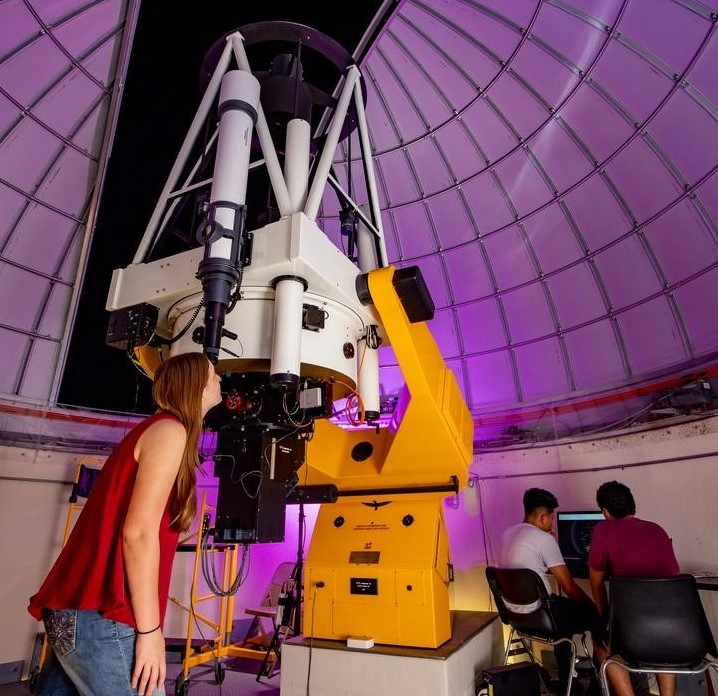## Aerospace, Physics, and Space Science Faculty Publications

### Observation of Z decays to four leptons with the CMS detector at the LHC

Article

#### Publication Title

Journal of High Energy Physics

#### Abstract

he first observation of the Z boson decaying to four leptons in proton-proton collisions is presented. The analyzed data set corresponds to an integrated luminosity of 5.02 fb−1 at s=7" role="presentation" style="box-sizing: inherit; display: inline; line-height: normal; word-spacing: normal; overflow-wrap: normal; text-wrap: nowrap; float: none; direction: ltr; max-width: none; max-height: none; min-width: 0px; min-height: 0px; border: 0px; padding: 0px; margin: 0px; position: relative;">s√=7�=7 TeV collected by the CMS detector at the Large Hadron Collider. A pronounced resonance peak, with a statistical significance of 9.7 σ, is observed in the distribution of the invariant mass of four leptons (electrons and/or muons) with mass and width consistent with expectations for Z boson decays. The branching fraction and cross section reported here are defined by phase space restrictions on the leptons, namely, 80 < m 4ℓ < 100 GeV, where m 4ℓ is the invariant mass of the four leptons, and m ℓℓ > 4 GeV for all pairs of leptons, where m ℓℓ is the two-lepton invariant mass. The measured branching fraction is B(Z→4ℓ)=(4.2−0.8+0.9(stat.)±0.2(syst.))×10−6" role="presentation" style="box-sizing: inherit; display: inline; line-height: normal; word-spacing: normal; overflow-wrap: normal; text-wrap: nowrap; float: none; direction: ltr; max-width: none; max-height: none; min-width: 0px; min-height: 0px; border: 0px; padding: 0px; margin: 0px; position: relative;">B(Z→4ℓ)=(4.2+0.9−0.8(stat.)±0.2(syst.))×10−6�(�→4ℓ)=(4.2−0.8+0.9(stat.)±0.2(syst.))×10−6 and agrees with the standard model prediction of 4.45 × 10−6. The measured cross section times branching fraction is σ(pp→Z)B(Z→4ℓ)=112−20+23(stat.)−5+7(syst.)−2+3(lumi.)" role="presentation" style="box-sizing: inherit; display: inline; line-height: normal; word-spacing: normal; overflow-wrap: normal; text-wrap: nowrap; float: none; direction: ltr; max-width: none; max-height: none; min-width: 0px; min-height: 0px; border: 0px; padding: 0px; margin: 0px; position: relative;">σ(pp→Z)B(Z→4ℓ)=112+23−20(stat.)+7−5(syst.)+3−2(lumi.)σ(pp→Z)�(Z→4ℓ)=112−20+23(stat.)−5+7(syst.)−2+3(lumi.) fb, also consistent with the standard model prediction of 120 fb. The four-lepton mass peak arising from Z → 4 decays provides a calibration channel for the Higgs boson search in the HZZ → 4 decay mode.

#### DOI

doi.org/10.1007/JHEP12(2012)034

2012

COinS